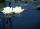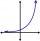# Sum-log

The sum of two numbers is 32, the sum of their logarithms (base 10) is 2.2. Determine these numbers.

Result

x =  25.875
y =  6.125

#### Solution:Leave us a comment of example and its solution (i.e. if it is still somewhat unclear...):

Showing 0 comments:Be the first to comment!#### To solve this verbal math problem are needed these knowledge from mathematics:

Looking for help with calculating roots of a quadratic equation? Do you have a linear equation or system of equations and looking for its solution? Or do you have quadratic equation?

## Next similar examples:

1. Exponential equationSolve exponential equation (in real numbers): 98x-2=9
2. Water liliesWater lilies are growing on the pond and their number is doubled every day. The whole layer is covered in 12 days. How many days will it cover 8 layers?
3. Ball gameRichard, Denis and Denise together scored 932 goals. Denis scored 4 goals over Denise but Denis scored 24 goals less than Richard. Determine the number of goals for each player.
4. Theorem proveWe want to prove the sentence: If the natural number n is divisible by six, then n is divisible by three. From what assumption we started?
5. EquationEquation ? has one root x1 = 8. Determine the coefficient b and the second root x2.
6. RootsDetermine the quadratic equation absolute coefficient q, that the equation has a real double root and the root x calculate: ?
7. DiscriminantDetermine the discriminant of the equation: ?
8. Powers 32 to the power of n divided by 4 to the power of -3 equal 4. What is the vaule of n?
9. Intercept with axisF(x)=log(x+4)-2, what is the x intercept
10. Logif ?, what is b?
11. Solve 3Solve quadratic equation: (6n+1) (4n-1) = 3n2
12. Quadratic equationFind the roots of the quadratic equation: 3x2-4x + (-4) = 0.
13. Cinema 4In cinema are 1656 seats and in the last row are 105 seats , in each next row 3 seats less. How many are the total rows in cinema?
14. Quadratic inequationIf 5x + x² > 100, then x is not
15. Men, women and childrenOn the trip went men, women and children in the ratio 2:3:5 by bus. Children pay 60 crowns and adults 150. How many women were on the bus when a bus was paid 4,200 crowns?
16. EquationHow many real roots has equation ? ?
17. Linsys2Solve two equations with two unknowns: 400x+120y=147.2 350x+200y=144